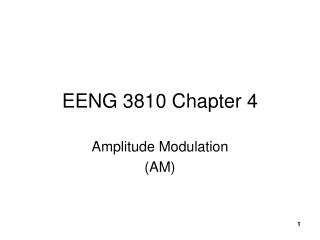DownloadDownload PresentationEENG 3810 Chapter 4

# EENG 3810 Chapter 4

Download Presentation## EENG 3810 Chapter 4

- - - - - - - - - - - - - - - - - - - - - - - - - - - E N D - - - - - - - - - - - - - - - - - - - - - - - - - - -
##### Presentation Transcript

1. EENG 3810 Chapter 4 Amplitude Modulation (AM) 1

2. Chapter 4 Homework 1. For an AM DSBFC modulator with a carrier frequency fc = 200KHz and a maximum modulating signal frequency fm(max) = 10 KHz, determine : a. Frequency limits for the upper and lower sidebands. b. Bandwidth. b. Upper and lower side frequencies produced when the modulating signal is a single-frequency 6 KHz tone. 2

3. Homework Continued 2. For the AM wave form above determine: 3

4. Homework Continued • Repeat steps (a) through (d) in Example 4 in these lecture slides for a modulation coefficient of 0.5. • For an AM DSBFC wave with a peak unmodulated carrier voltage Vc = 20 Vp, a load resistance RL = 20 , and a modulation coefficient m = 0.8, determine the power of the modulated wave 5

5. Homework Continued 6.Determine the noise improvement for a receiver with an RF bandwidth equal to 100 KHz and an IF bandwidth equal to 20 KHz.

6. Frequency Spectrum of An AM Double Sideband Full Carrier (DSBFC) Wave 9

7. Example 1 For an AM DSBFC modulator with a carrier frequency fc = 100KHz and a maximum modulating signal frequency fm(max) = 5 KHz, determine : a. Frequency limits for the upper and lower sidebands. b. Bandwidth. c. Upper and lower side frequencies produced when the modulating signal is a single-frequency 3 KHz tone. 10

8. Example 1 Solution a. b. c. 11

9. Phasor addition in an AM DSBFC envelope • For a single-frequency modulating signal, am AM envelop is produced from the vector addition of the carrier and upper and lower side frequencies. Phasors of the carrier, • The upper and lower frequencies combine and produce a resultant component that combines with the carrier component. • Phasors for the carrier, upper and lower frequencies all rotate in the counterclockwise direction. • The upper sideband frequency rotates faster than the carrier. (usf> c) • The lower sideband frequency rotes slower than the carrier. (usf< c) 13

10. If the modulating signal is pure, single frequency sine wave and the modulation process is symmetrical, the % modulation can be derived as follows: 16

11. Peak Amplitudes of Upper and Lower Sidebands The peak change in amplitude of the output wave (Em) is equal to the sum of the voltages from the upper and lower sideband frequencies. Therefore, 17

12. Percent Modulation of An AM DSBFC Envelope(a) modulating signal; (b) unmodulated carrier; (c) 50% modulated wave; (d) 100% modulated wave 18

13. Example 2 For the AM wave form above determine: 19

14. Generation of an AM DSBFC Envelope Shown in The Time Domain –½ cos(230t) sin(225t) + ½ cos(220t) summation of (a), (b), and (c) 22

15. Power Spectrum for an AM DSBFC Wave with a Single-frequency Modulating Signal 30

16. Single-Sideband

17. Conventional DSFC-AM

18. Single-side Band Full Carrier (SSBFC) The carrier is transmitted at full power and only one sideband is transmitted.

19. SSBFC waveform, 100% modulation

20. Single-Sideband Suppressed Carrier (SSBSC) The carrier is suppressed 100% and one sideband is removed. Only one sideband is transmitted.

21. SSBSC waveform

22. Single-Sideband Reduced Carrier(SSBRC) One sideband is removed and the carrier voltage is reduced to 10% of its un-modulated amplitude.

23. Independent Sideband(ISB) A single carrier is independently modulated by two different modulating signals.

24. ISB waveform

25. Vestigial Sideband(VSB) The carrier and one complete sideband are transmitted, but only part of the other sideband is transmitted.